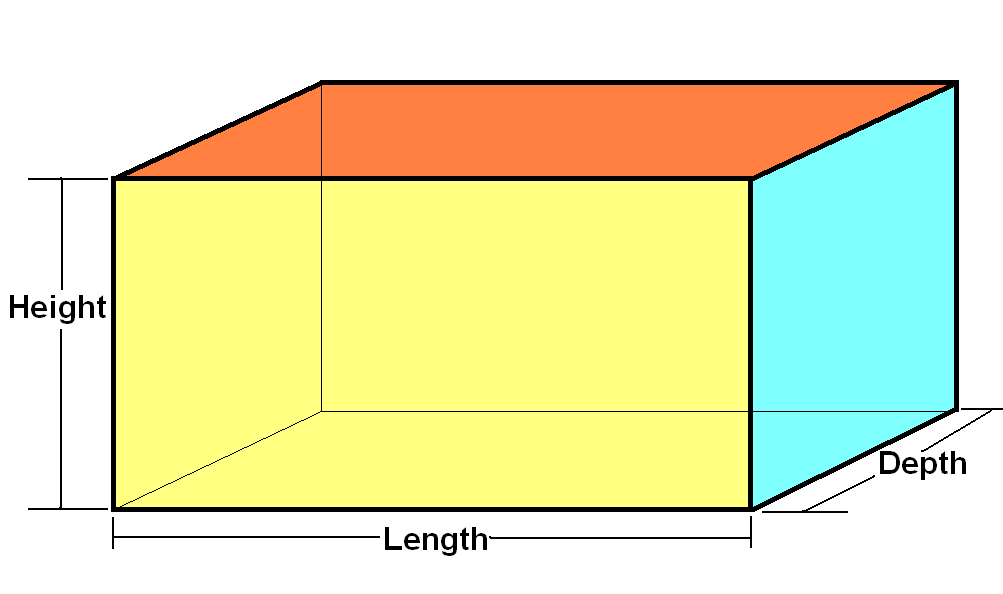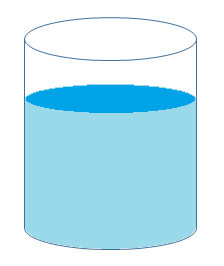# Volume and Capacity

Volume and Capacity: The measurement of the total space occupied by a solid is the volume of a three-dimensional figure. Any object that has length, breadth, and thickness is a three-dimensional figure. The difference between the total amount of space left inside the hollow body and the space occupied by the body is the volume of a hollow 3-dimensional figure. Now let’s talk about capacity. As we know that there are many hollow objects that we come across in our daily life. Capacity (with reference to volume) may be defined as the maximum amount of matter that an object can hold.

Some of the units for measuring volume are cm3, m3, litre, millilitre etc.

Let us take the example of a 3d shape called cuboid, it is a solid bounded by six rectangles and thus has six rectangular faces. The dimensions of the cuboid are as follows: length l, breadth b and height h.

Volume of the cuboid is given by the formula:

V = l × b × h## What is Capacity?

The hollow objects are filled with air or liquid which take the shape of the container. The total volume of air and water that is accumulated inside a container is the capacity of that container. We can still distinguish between volume and capacity even after many similarities. When dealing with capacity, more concentration is on how much solid, liquid or gas can fit into the container and it also implies the bearing power of that container. We can measure capacity in cm3, m3, litres, millilitres, pounds, gallons etc.In the above figure, the cylinder is filled with water to a certain extent. Here, the amount of water that the cylinder can hold is referred to as the capacity of the cylinder. However, to find the capacity of cylinder one can use the formula of volume of a cylinder.

## Differences between Volume and Capacity

Both volume and capacity are considered somewhat similar but there are some differences between them that make them different.

• The total amount of any substance which is contained in a particular space is called the volume. The total potential amount of any substance that can be contained in a space is called the capacity of that space.

This can be summarised as shown in the table below.

 Volume Capacity Volume indicates the total amount of space covered by an object in three-dimensional space. Capacity refers to the ability of something (like a solid substance, gas or liquid) to hold, absorb or receive by an object. Common units (units of measurement) – cm3, m3 Common units (units of measurement)- litre, gallons, pounds Both solid and hollow objects have volume. Only hollow objects have the capacity. Example – Cube, Cuboid, Cone and Cylinder Example – Cone, Cylinder, hollow hemisphere

This can be well understood with the help of a solved example given below.

Example: Find the volume and capacity (in litres) of a cylinder whose radius is 7 cm and height is 20 cm.

Solution:

Given, r = 7 cm and h = 20 cm

We know that,

Volume of cylinder = πr2h

= (22/7) × 7 × 7 × 20

= 22 × 7 × 20

= 3080 cm3

Capacity of the cylinder = 3080/1000 litres (1000 cm3 = 1 litre)

= 3.08 litres

Note: We can also represent capacity in terms of cm3. Hence, in the above example volume will be numerically equal to capacity.

## Frequently Asked Questions – FAQs

Q1

### What is the difference between Area, volume and capacity?

Area is the space occupied in a 2-Dimensional plane. It can be measured in x-y coordinates.
Volume is the space occupied in a 3-Dimensional plane. It can be measured in x-y-z coordinates
Capacity is the measurement of how much solid/liquid/gas can be held in a volume.
Q2

### What are the units of Volume and capacity?

Unit for volume is cubic meter = m3
Unit for capacity is liters = L.
Q3

### How to measure volume?

Volume is measured as the product of length, breadth and height.
i,e V = L × B × H or v = l × b × h
Q4

### Why do we use Volume and capacity?

By determining the volume of an object, we can identify the amount required to fill that object. For example, Filling water in a bucket or overhead tank.
Q5

### Give an example for Volume and capacity.

Example: Sump filled with water. The volume of the sump will be the product of its dimensions, length × breadth × depth, where as the amount of water forms the capacity